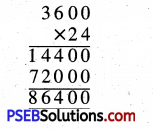# PSEB 5th Class Maths Solutions Chapter 2 Fundamental Operations on Numbers Ex 2.6

Punjab State Board PSEB 5th Class Maths Book Solutions Chapter 2 Fundamental Operations on Numbers Ex 2.6 Textbook Exercise Questions and Answers.

## PSEB Solutions for Class 5 Maths Chapter 2 Fundamental Operations on Numbers Ex 2.6

Question 1.
The price of a cycle is ₹ 5699. What is price of 17 cycles ?
Solution:
Cost price of 1 cycle = ₹ 5699
Cost price of 17 cycles = ₹ 5699 × 17
= ₹ 96883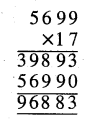Question 2.
There are 12 tiles in a box. How many tiles are there in 4590 boxes ?
Solution:
Number of tiles in one box = 12
Number of tiles in 4590 in boxes = 4590 × 12 = 55080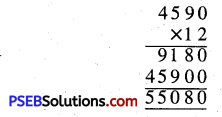Question 3.
Multiply 4 digit smallest number with 98.
Solution:
Smallest 4 digit number = 1000
Requires product = 1000 × 98 = 98000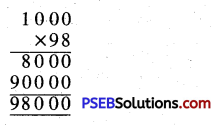Question 4.
Rate list of electrical equipment in electrical shop is as follows :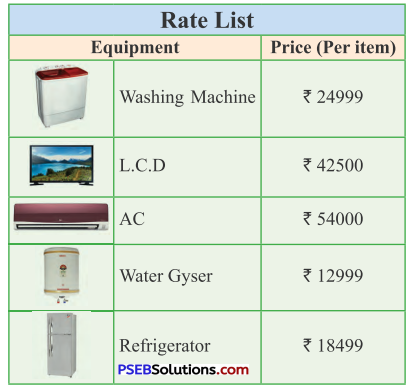(i) Charan has ₹ 1 lakh with him. He buys 2 washing machines and one L.C.D. How much amount has he spent ?
(ii) Charan’s brother has ₹ 1 lakh. He buys one A.C., Two water Gysers and one Refrigerator. How much amount is left with him ?
Solution:
(i) Cost price of 1 washing machine
= ₹ 24999
Cost price of 2 washing machines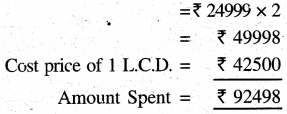(ii) Cost price of 1 Water Gyser = ₹ 12999
Cost price of 2 Water Gyser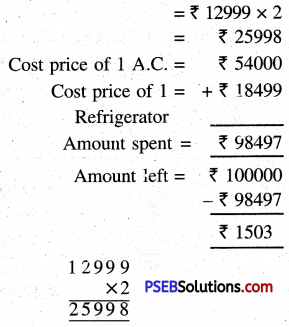Question 5.
A factory manufactures 4990 toffees a day. How many toffees will be manufactured in 19 days ?
Solution:
Number of toffees manufactured in 1 day = 4990
Number of toffees manufactured in 19 days = 4990 × 19 = 94810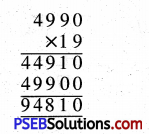Question 6.
6798 bricks are loaded in a tractor in an hour. How many bricks will be loaded in 13 hours ?
Solution:
Number of bricks loaded in one hour = 6798
Number of bricks loaded in 13 hours = 6798 × 13 = 88374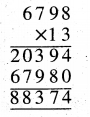Question 7.
A shopkeeper sells one mobile phone for t 5089. If he sells 18 such mobile phones in a day, how much amount would he collect in a day ?
Solution:
Selling price of 1 mobile phone = ₹ 5089
Selling price of 18 mobile phones = ₹ 5089 × 18
The amount he will collect in one day
= ₹ 91602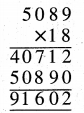Question 8.
Multiply 3 digit largest number with 95.
Solution:
Greatest number of three digits
= 999
Required product = 999 × 95 = 94905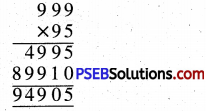Question 9.
How many seconds are there in 24 hours ?
Solution:
Number of seconds in one hour
= 3600
Number of seconds in 24 hours = 3600 × 24 = 86400